# Question?

Hello!!!

My name is Waleria. I
work at INPE in Sao Jose dos Campos, Brazil. And I’d like to make a question. I’m in trouble to generate a two functions graph.

I have a problem to generate a graph of the two functions. I have this functions, is bellow:

``````__y1 = -108*(x**2)/(3e14**2)__
__y2 = 1*((1.38e-23*(1e0+4)/1e-6)*((x**2)/4*(1e4**2)*(3e14**2))))__
However, i need to plot a graph these two functions, the first function is decrescent an the second is crescent. I made some tests that cite below:
1 - I plotted only function at a time and the result was, the result of the first test is attached;
2- Then, I see the example below: [http://matplotlib.sourceforge.net/examples/pylab_examples/set_and_get.html](http://matplotlib.sourceforge.net/examples/pylab_examples/set_and_get.html)
So, I made a change in the example over, I changed the functions in the example and added my functions as falows below:
__from pylab import *
x = arange(3000,3400)
y1 = -108*(x**2)/(3e14**2)
y2 = 1*((1.38e-23*(1e0+4)/1e-6)*((x**2)/4*(1e4**2)*(3e14**2)))
lines = plot(x, y1, x, y2)__

However, the result in my opinion is incorrect, because the first function is decrescent and second is crescent, see the result of second test, is attached.

As can see in the image, first appers a continuous line and to ber a constant function should be of the type y=x for the example, the second function is crescent, ou seja, the first function is incorrect, i gave a print in the

functions y1 and y2, the output values are correct, for function y1 the values are always decrescent and function(negative values) and for y2 the values are crescent ( positive values).

As you

can see in the image first appears a continuous line, and to be a
constant function should be of the type y = x for example, the second
function is increasing, ie, the first function is being displayed
incorrectly.

Already relize tests using GNUPLOT and the results is equal to the tests over.

I would like to thank your support.
Best regards
``````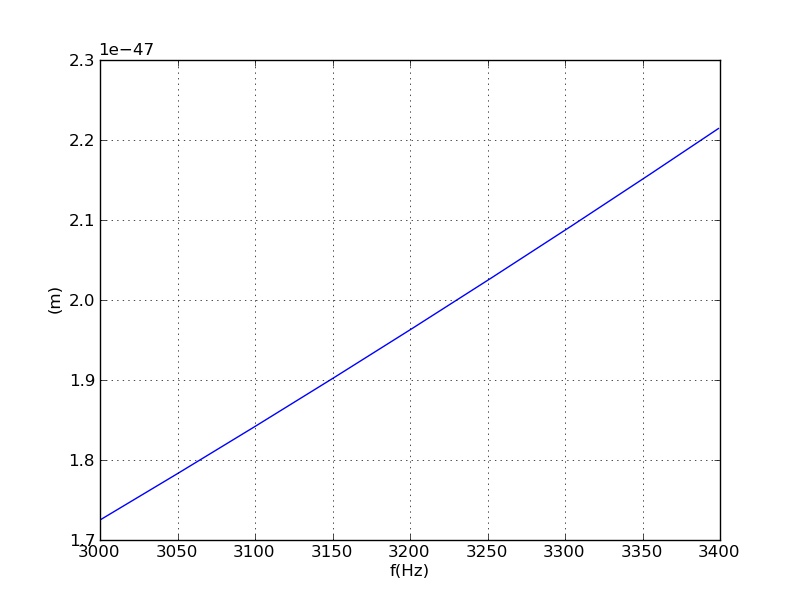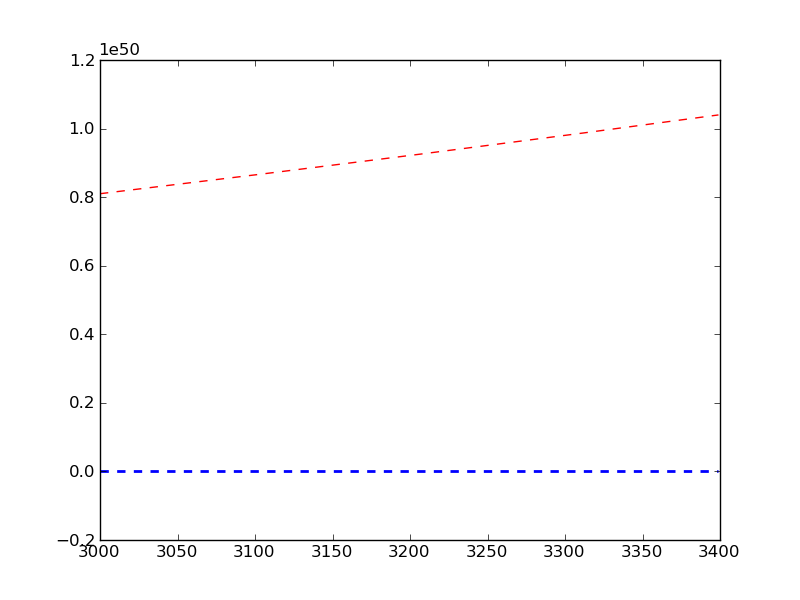I tried to reproduce your attached plots using the functions you gave. While I was able to reproduce your first graph (values from -1.08e-20 to -1.386e-20) I could not reproduce your second graph. My y2 has values from 1.397e27 to 1.7936e27. Your second graph shows values ranging from 1.7e-27 to 2.2e-27.
As for your third graph, I am not sure where that came from, but the red line has values around 1e50 which is nowhere near the values of your other two graphs.
y1 seems to be the only one that is correct here. Could it be that the y2 function was typed incorrectly somewhere? I noticed some of the notation is awkward, for example "(1e0+4)", shouldn’t that simpilfy to 5?

I hope this helps,
Ben Root

···

On Tue, Jun 8, 2010 at 10:19 AM, Waléria Antunes David <waleriantunes@…287…> wrote:

Hello!!!

My name is Waleria. I
work at INPE in Sao Jose dos Campos, Brazil. And I’d like to make a question. I’m in trouble to generate a two functions graph.

I have a problem to generate a graph of the two functions. I have this functions, is bellow:

``````__y1 = -108*(x**2)/(3e14**2)__
__y2 = 1*((1.38e-23*(1e0+4)/1e-6)*((x**2)/4*(1e4**2)*(3e14**2))))__
However, i need to plot a graph these two functions, the first function is decrescent an the second is crescent. I made some tests that cite below:
1 - I plotted only function at a time and the result was, the result of the first test is attached;
2- Then, I see the example below: [http://matplotlib.sourceforge.net/examples/pylab_examples/set_and_get.html](http://matplotlib.sourceforge.net/examples/pylab_examples/set_and_get.html)
So, I made a change in the example over, I changed the functions in the example and added my functions as falows below:
__from pylab import *
x = arange(3000,3400)
y1 = -108*(x**2)/(3e14**2)
y2 = 1*((1.38e-23*(1e0+4)/1e-6)*((x**2)/4*(1e4**2)*(3e14**2)))
lines = plot(x, y1, x, y2)__

However, the result in my opinion is incorrect, because the first function is decrescent and second is crescent, see the result of second test, is attached.

As can see in the image, first appers a continuous line and to ber a constant function should be of the type y=x for the example, the second function is crescent, ou seja, the first function is incorrect, i gave a print in the

functions y1 and y2, the output values are correct, for function y1 the values are always decrescent and function(negative values) and for y2 the values are crescent ( positive values).

As you

can see in the image first appears a continuous line, and to be a
constant function should be of the type y = x for example, the second
function is increasing, ie, the first function is being displayed
incorrectly.

Already relize tests using GNUPLOT and the results is equal to the tests over.

I would like to thank your support.
Best regards
``````

ThinkGeek and WIRED’s GeekDad team up for the Ultimate

GeekDad Father’s Day Giveaway. ONE MASSIVE PRIZE to the

lucky parental unit. See the prize list and enter to win:

http://p.sf.net/sfu/thinkgeek-promo

Matplotlib-users mailing list

Matplotlib-users@lists.sourceforge.net

https://lists.sourceforge.net/lists/listinfo/matplotlib-users

Waléria Antunes David wrote:

Hello!!!

My name is Waleria. I work at INPE in Sao Jose dos Campos, Brazil. And
I’d like to make a question. I’m in trouble to generate a two functions
graph.

I have a problem to generate a graph of the two functions. I have this
functions, is bellow:

``````__y1 = -108*(x**2)/(3e14**2)__
__y2 = 1*((1.38e-23*(1e0+4)/1e-6)*((x**2)/4*(1e4**2)*(3e14**2))))__
However, i need to plot a graph these two functions, the first function is
decrescent an the second is crescent. I made some tests that cite below:
1 - I plotted only function at a time and the result was, the result of the first test is attached;
2- Then, I see the example below: [http://matplotlib.sourceforge.net/examples/pylab_examples/set_and_get.html](http://matplotlib.sourceforge.net/examples/pylab_examples/set_and_get.html)
So, I made a change in the example over, I changed the functions in the example and added my functions as falows below:
__
from pylab import *
x = arange(3000,3400)
y1 = -108*(x**2)/(3e14**2)
y2 = 1*((1.38e-23*(1e0+4)/1e-6)*((x**2)/4*(1e4**2)*(3e14**2)))
lines = plot(x, y1, x, y2)__

However, the result in my opinion is incorrect, because the first function is decrescent and second is crescent, see the result of second test, is attached.
As can see in the image, first appers a continuous line and to ber a constant function should be of the type y=x for the example, the second function is crescent, ou seja, the first function is incorrect, i gave a print in the functions y1 and y2, the output values are correct, for function y1 the values are always decrescent and function(negative values) and for y2 the values are crescent ( positive values).
As you can see in the image first appears a continuous line, and to be a constant function should be of the type y = x for example, the second function is increasing, ie, the first function is being displayed incorrectly.

Already relize tests using GNUPLOT and the results is equal to the tests over.
I would like to thank your support.
Best regards
``````

Hello Waleria,

I’m not clear on what you are expecting to see in your plots.

I added print statements, for y1 and y2, to your code and the results
are:

• y1 min and max: -1.38638412e-20 -1.08e-20
• y2 min and max: 1.39725e+27 1.7936343e+27
So your first plot appears to be correct.

Plotting y2 and y1, on the same (linear) figure will show y1 to be
essentially zero because y2 is 27 orders of magnitude greater.

What am I misunderstanding?

– jv

···

You might need to check your y2. You are mixing integers and floats which possible have resulted with some rounding errors. I get e+30 when I assert the terms as floats in y2.

For the plotting: y1 is around -e-20 whereas y2 goes up to e+30. You can’t see the trend easily on one axes even if you could scale them logarithmically (hint logarithm is only defined for positive numbers). You can make a positive assumption for the y1 and plot them on one y-axes

yscale(‘log’)

axis(ymin=1e+20, ymax=1e+30)

Still this won’t let you see the functions trends that you are looking to see. I would suggest you to use multipe figures or investing a bit more time to use parasite axes example of JJ.

···

On Tue, Jun 8, 2010 at 10:19 AM, Waléria Antunes David <waleriantunes@…287…> wrote:

Hello!!!

My name is Waleria. I
work at INPE in Sao Jose dos Campos, Brazil. And I’d like to make a question. I’m in trouble to generate a two functions graph.

I have a problem to generate a graph of the two functions. I have this functions, is bellow:

``````__y1 = -108*(x**2)/(3e14**2)__
__y2 = 1*((1.38e-23*(1e0+4)/1e-6)*((x**2)/4*(1e4**2)*(3e14**2))))__

``````

Gökhan

Decreasing and increasing over the specified range, you mean.
You won't see that when you plot them together
because they have very different scales,
so the one with the small scale will look flat.

Alan Isaac

PS I suspect you did integer division without intending to.
Here's a fix for that. You might also want to check your
parentheses, noting 1.0/2*4 is 2 (not 1/8), but I assumed

import numpy as np
import matplotlib.pyplot as plt

x = np.arange(3000,3400, dtype=np.float) #use floats; not integers!

x2 = x*x
scale1 = -108 / 3e14**2

y1 = scale1 * x2

scale2 = 1*((1.38e-23*(1e0+4)/1e-6)*(1.0/4*(1e4**2)*(3e14**2)))
scale2b = 1.38e19 * 11.25 #more accurate; less costly
print scale2, scale2b, scale2 - scale2b # unnecessary error
y2 = scale2b * x2

fig = plt.figure()
ax.plot(x,y1)
ax.plot(x,y2)

fig = plt.figure()
ax.plot(x, y1, label='y1')
ax.plot(x, y2, label='y2')
ax.legend()

plt.show()

···

On 6/8/2010 11:19 AM, Wal�ria Antunes David wrote:

the first function isdecrescent an the second is crescent

Decreasing and increasing over the specified range, you mean.
You won't see that when you plot them together
because they have very different scales,
so the one with the small scale will look flat.

Alan Isaac

PS I suspect you did integer division without intending to.
Here's a fix for that. You might also want to check your
parentheses, noting 1.0/2*4 is 2 (not 1/8), but I assumed

PPS Sorry if this is sent twice ...

import numpy as np
import matplotlib.pyplot as plt

x = np.arange(3000,3400, dtype=np.float) #use floats; not integers!

x2 = x*x
scale1 = -108 / 3e14**2

y1 = scale1 * x2

scale2 = 1*((1.38e-23*(1e0+4)/1e-6)*(1.0/4*(1e4**2)*(3e14**2)))
scale2b = 1.38e19 * 11.25 #more accurate; less costly
print scale2, scale2b, scale2 - scale2b # unnecessary error
y2 = scale2b * x2

fig = plt.figure()
ax.plot(x,y1)
ax.plot(x,y2)

fig = plt.figure()
ax.plot(x, y1, label='y1')
ax.plot(x, y2, label='y2')
ax.legend()

plt.show()

···

On 6/8/2010 11:19 AM, Wal�ria Antunes David wrote:

the first function isdecrescent an the second is crescent

``````I understand what you are saying, but my functions are these, attached.

Scientific notation in Python is not so?
**Math**               **Python**

Pinc = 10<sup>-6      </sup>Pinc = 1e-6

3,0x10<sup>14</sup>        3e14

?

Then, changing my functions of the images attached for python is as bellow:

``````

y1 = -108*(x2)/(3e142)

y2 = 1*((1.38e-23*(1e0+4)/1e-6)((x**2)/4(1e42)*(3e142))))

Is not it?

``````

<details class='elided'>
<summary title='Show trimmed content'>&#183;&#183;&#183;</summary>

On Tue, Jun 8, 2010 at 1:34 PM, Gökhan Sever <gokhansever@...287...> wrote:
>
>
> On Tue, Jun 8, 2010 at 10:19 AM, Waléria Antunes David <waleriantunes@...287...> wrote:

> >  Hello!!!
> >
> >
> > My name is Waleria. I
> > work at INPE in Sao Jose dos Campos, Brazil. And I'd like to make a question. I'm in trouble to generate a two functions graph.
> >
> >
> >
> > I have a problem to generate a graph of the two functions. I have this functions, is bellow:
> >
> >
> > ```
> > __y1 = -108*(x**2)/(3e14**2)__
> > __y2 = 1*((1.38e-23*(1e0+4)/1e-6)*((x**2)/4*(1e4**2)*(3e14**2))))__
> >
> >
> >
> > ```

> You might need to check your y2. You are mixing integers and floats which possible have resulted with some rounding errors. I get e+30 when I assert the terms as floats in y2.

>

> For the plotting: y1 is around -e-20 whereas y2 goes up to e+30. You can't see the trend easily on one axes even if you could scale them logarithmically (hint logarithm is only defined for positive numbers). You can make a positive assumption for the y1 and plot them on one y-axes

>

> yscale('log')

> axis(ymin=1e+20, ymax=1e+30)

>

> Still this won't let you see the functions trends that you are looking to see.  I would suggest you to use multipe figures or investing a bit more time to use parasite axes example of JJ.

>

>

>

>
> --
> Gökhan
>

</details>``````

So my assumption in my previous post that you had parentheses where
you wanted them was wrong. (See the post.) Also, see my posted
example explaining G�khan's point. (He is correct.)

hth,
Alan Isaac

···

On Tue, Jun 8, 2010 at 1:34 PM, G�khan Sever <gokhansever@...287...> wrote:
> You might need to check your y2. You are mixing integers and floats
> which possible have resulted with some rounding errors. I get e+30
> when I assert the terms as floats in y2.

On 6/8/2010 1:47 PM, Wal�ria Antunes David wrote:

I understand what you are saying, but my functions are these, attached.

I think I found your errors.
First, you are correct, the scientific notation in python is as you understand, there is nothing wrong with that.
Instead, you have a problem with order of operations. In the last quantity, you have "((x2)/4*(1e42)(3e142))"
According to the equation that you wrote out in the attached image, you need: "((x
2)/*(4(2e42)
(3e142)))"
Note that you needed parentheses for the entire denominator. Also, the 1e4
2 should have been 2e4**2.

This gives me values between 4.3125e-48 and 5.5359e-48.

Ben Root

···

On Tue, Jun 8, 2010 at 12:47 PM, Waléria Antunes David <waleriantunes@…287…> wrote:

``````I understand what you are saying, but my functions are these, attached.

Scientific notation in Python is not so?
**Math**               **Python**
``````

Pinc = 10-6 Pinc = 1e-6

3,0x1014 3e14

?

Then, changing my functions of the images attached for python is as bellow:

``````y1 = -108*(x**2)/(3e14**2)

y2 = 1*((1.38e-23*(1e0+4)/1e-6)*((x**2)/4*(1e4**2)*(3e14**2))))

``````

Is not it?

On Tue, Jun 8, 2010 at 1:34 PM, Gökhan Sever <gokhansever@…83…287…> wrote:

On Tue, Jun 8, 2010 at 10:19 AM, Waléria Antunes David <waleriantunes@…287…> wrote:

Hello!!!

My name is Waleria. I
work at INPE in Sao Jose dos Campos, Brazil. And I’d like to make a question. I’m in trouble to generate a two functions graph.

I have a problem to generate a graph of the two functions. I have this functions, is bellow:

``````__y1 = -108*(x**2)/(3e14**2)__
__y2 = 1*((1.38e-23*(1e0+4)/1e-6)*((x**2)/4*(1e4**2)*(3e14**2))))__

``````

You might need to check your y2. You are mixing integers and floats which possible have resulted with some rounding errors. I get e+30 when I assert the terms as floats in y2.

For the plotting: y1 is around -e-20 whereas y2 goes up to e+30. You can’t see the trend easily on one axes even if you could scale them logarithmically (hint logarithm is only defined for positive numbers). You can make a positive assumption for the y1 and plot them on one y-axes

yscale(‘log’)

axis(ymin=1e+20, ymax=1e+30)

Still this won’t let you see the functions trends that you are looking to see. I would suggest you to use multipe figures or investing a bit more time to use parasite axes example of JJ.

Gökhan

ThinkGeek and WIRED’s GeekDad team up for the Ultimate

GeekDad Father’s Day Giveaway. ONE MASSIVE PRIZE to the

lucky parental unit. See the prize list and enter to win:

http://p.sf.net/sfu/thinkgeek-promo

Matplotlib-users mailing list

Matplotlib-users@lists.sourceforge.net

https://lists.sourceforge.net/lists/listinfo/matplotlib-users

no, I think, did not understand my functions…see…i made a change my second function in the attached.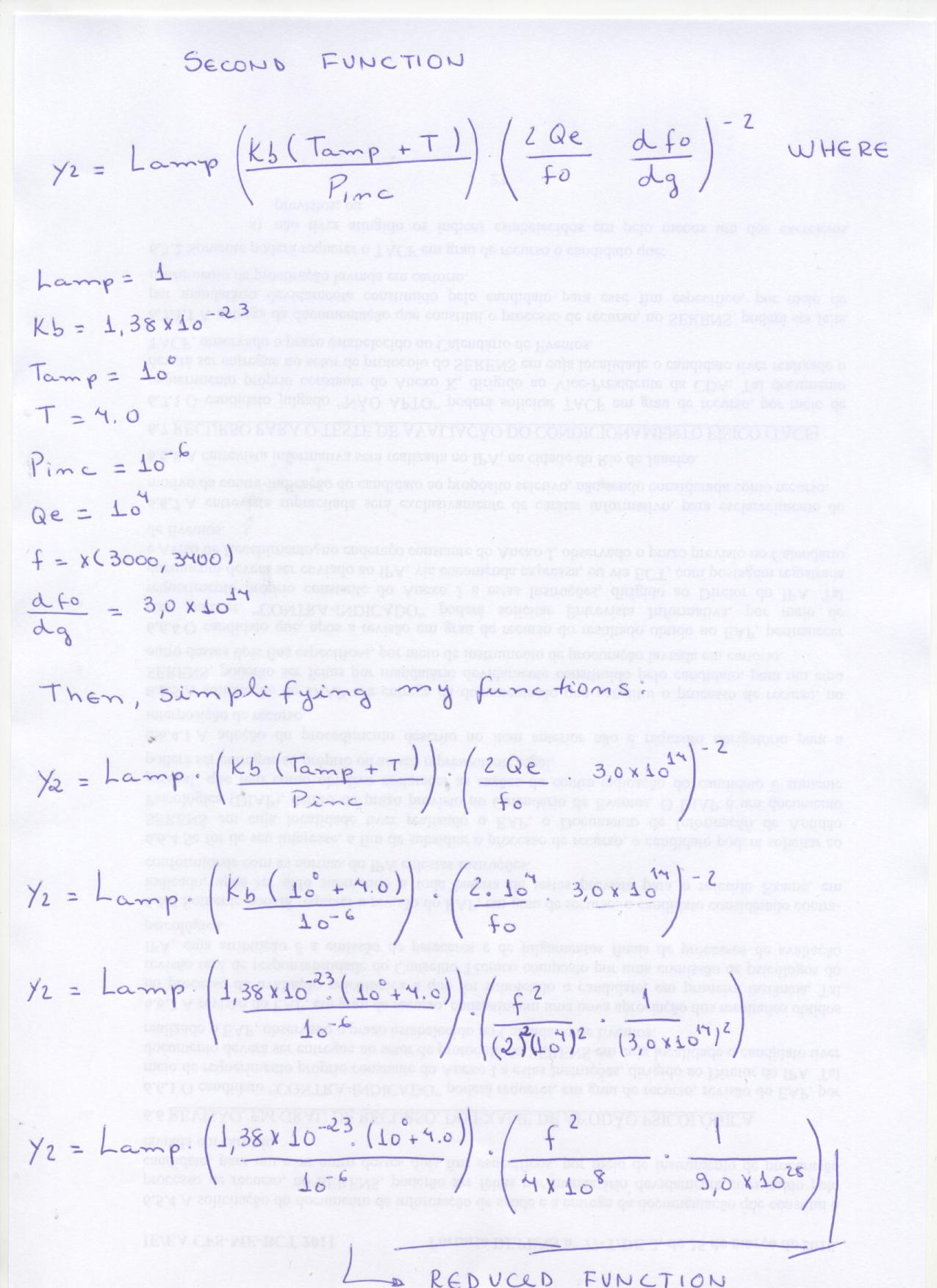···

On Tue, Jun 8, 2010 at 3:48 PM, Benjamin Root <ben.root@…1304…> wrote:

I think I found your errors.
First, you are correct, the scientific notation in python is as you understand, there is nothing wrong with that.
Instead, you have a problem with order of operations. In the last quantity, you have "((x2)/4*(1e42)(3e142))"
According to the equation that you wrote out in the attached image, you need: "((x
2)/*(4(2e42)
(3e142)))"
Note that you needed parentheses for the entire denominator. Also, the 1e4
2 should have been 2e4**2.

This gives me values between 4.3125e-48 and 5.5359e-48.

Ben Root

On Tue, Jun 8, 2010 at 12:47 PM, Waléria Antunes David <waleriantunes@…287…> wrote:

``````I understand what you are saying, but my functions are these, attached.

Scientific notation in Python is not so?
**Math**               **Python**
``````

Pinc = 10-6 Pinc = 1e-6

3,0x1014 3e14

?

Then, changing my functions of the images attached for python is as bellow:

``````y1 = -108*(x**2)/(3e14**2)

y2 = 1*((1.38e-23*(1e0+4)/1e-6)*((x**2)/4*(1e4**2)*(3e14**2))))

``````

Is not it?

On Tue, Jun 8, 2010 at 1:34 PM, Gökhan Sever <gokhansever@…287…> wrote:

On Tue, Jun 8, 2010 at 10:19 AM, Waléria Antunes David <waleriantunes@…287…> wrote:

Hello!!!

My name is Waleria. I
work at INPE in Sao Jose dos Campos, Brazil. And I’d like to make a question. I’m in trouble to generate a two functions graph.

I have a problem to generate a graph of the two functions. I have this functions, is bellow:

``````__y1 = -108*(x**2)/(3e14**2)__
__y2 = 1*((1.38e-23*(1e0+4)/1e-6)*((x**2)/4*(1e4**2)*(3e14**2))))__

``````

You might need to check your y2. You are mixing integers and floats which possible have resulted with some rounding errors. I get e+30 when I assert the terms as floats in y2.

For the plotting: y1 is around -e-20 whereas y2 goes up to e+30. You can’t see the trend easily on one axes even if you could scale them logarithmically (hint logarithm is only defined for positive numbers). You can make a positive assumption for the y1 and plot them on one y-axes

yscale(‘log’)

axis(ymin=1e+20, ymax=1e+30)

Still this won’t let you see the functions trends that you are looking to see. I would suggest you to use multipe figures or investing a bit more time to use parasite axes example of JJ.

Gökhan

ThinkGeek and WIRED’s GeekDad team up for the Ultimate

GeekDad Father’s Day Giveaway. ONE MASSIVE PRIZE to the

lucky parental unit. See the prize list and enter to win:

http://p.sf.net/sfu/thinkgeek-promo

Matplotlib-users mailing list

Matplotlib-users@lists.sourceforge.net

https://lists.sourceforge.net/lists/listinfo/matplotlib-users

You can further simplify your function. Try grouping 10 powers under one term. You can then Pythonify your function (less terms to write less prone to typing errors). Actually, if you previously define your terms before the function definition you don’t need any simplifications or such.

···

On Tue, Jun 8, 2010 at 2:05 PM, Waléria Antunes David <waleriantunes@…287…> wrote:

no, I think, did not understand my functions…see…i made a change my second function in the attached.

Gökhan

Waléria,

I repeat, you need to add parentheses for the denominator of that quantity in order to match what you wrote out on paper. You are missing a set of parentheses that is causing (3e14**2) to be multiplied rather than divided. This explains the huge values you are getting.

Ben Root

···

On Tue, Jun 8, 2010 at 2:05 PM, Waléria Antunes David <waleriantunes@…287…> wrote:

no, I think, did not understand my functions…see…i made a change my second function in the attached.

On Tue, Jun 8, 2010 at 3:48 PM, Benjamin Root <ben.root@…1304…> wrote:

I think I found your errors.
First, you are correct, the scientific notation in python is as you understand, there is nothing wrong with that.
Instead, you have a problem with order of operations. In the last quantity, you have "((x2)/4*(1e42)(3e142))"
According to the equation that you wrote out in the attached image, you need: "((x
2)/*(4(2e42)
(3e142)))"
Note that you needed parentheses for the entire denominator. Also, the 1e4
2 should have been 2e4**2.

This gives me values between 4.3125e-48 and 5.5359e-48.

Ben Root

On Tue, Jun 8, 2010 at 12:47 PM, Waléria Antunes David <waleriantunes@…287…> wrote:

``````I understand what you are saying, but my functions are these, attached.

Scientific notation in Python is not so?
**Math**               **Python**
``````

Pinc = 10-6 Pinc = 1e-6

3,0x1014 3e14

?

Then, changing my functions of the images attached for python is as bellow:

``````y1 = -108*(x**2)/(3e14**2)

y2 = 1*((1.38e-23*(1e0+4)/1e-6)*((x**2)/4*(1e4**2)*(3e14**2))))

``````

Is not it?

On Tue, Jun 8, 2010 at 1:34 PM, Gökhan Sever <gokhansever@…287…> wrote:

On Tue, Jun 8, 2010 at 10:19 AM, Waléria Antunes David <waleriantunes@…287…> wrote:

Hello!!!

My name is Waleria. I
work at INPE in Sao Jose dos Campos, Brazil. And I’d like to make a question. I’m in trouble to generate a two functions graph.

I have a problem to generate a graph of the two functions. I have this functions, is bellow:

``````__y1 = -108*(x**2)/(3e14**2)__
__y2 = 1*((1.38e-23*(1e0+4)/1e-6)*((x**2)/4*(1e4**2)*(3e14**2))))__

``````

You might need to check your y2. You are mixing integers and floats which possible have resulted with some rounding errors. I get e+30 when I assert the terms as floats in y2.

For the plotting: y1 is around -e-20 whereas y2 goes up to e+30. You can’t see the trend easily on one axes even if you could scale them logarithmically (hint logarithm is only defined for positive numbers). You can make a positive assumption for the y1 and plot them on one y-axes

yscale(‘log’)

axis(ymin=1e+20, ymax=1e+30)

Still this won’t let you see the functions trends that you are looking to see. I would suggest you to use multipe figures or investing a bit more time to use parasite axes example of JJ.

Gökhan

ThinkGeek and WIRED’s GeekDad team up for the Ultimate

GeekDad Father’s Day Giveaway. ONE MASSIVE PRIZE to the

lucky parental unit. See the prize list and enter to win:

http://p.sf.net/sfu/thinkgeek-promo

Matplotlib-users mailing list

Matplotlib-users@lists.sourceforge.net

https://lists.sourceforge.net/lists/listinfo/matplotlib-users

Hello…

Benjamin,

I made a correction in my functions, i added parentheses for the denominator in the functions, saw the images in the attached:

two_functions.jpg
result in graph = images_.png
output values = values of the first function and values of the second function

Is there a way to plot my functions in the graph so that one can to visualize the difference between them.

This is correct my functions?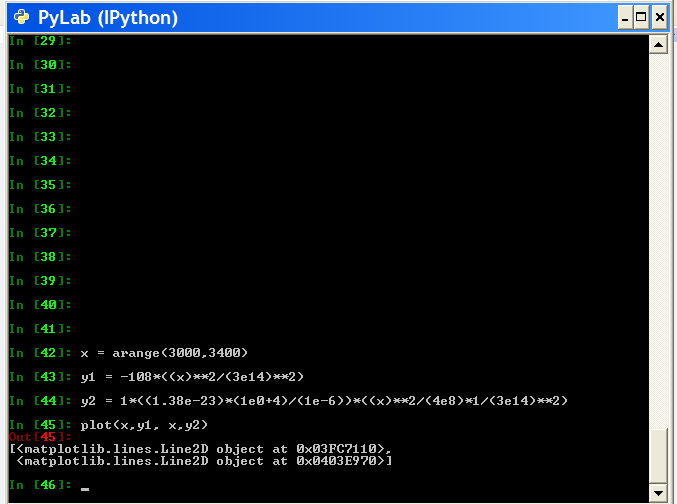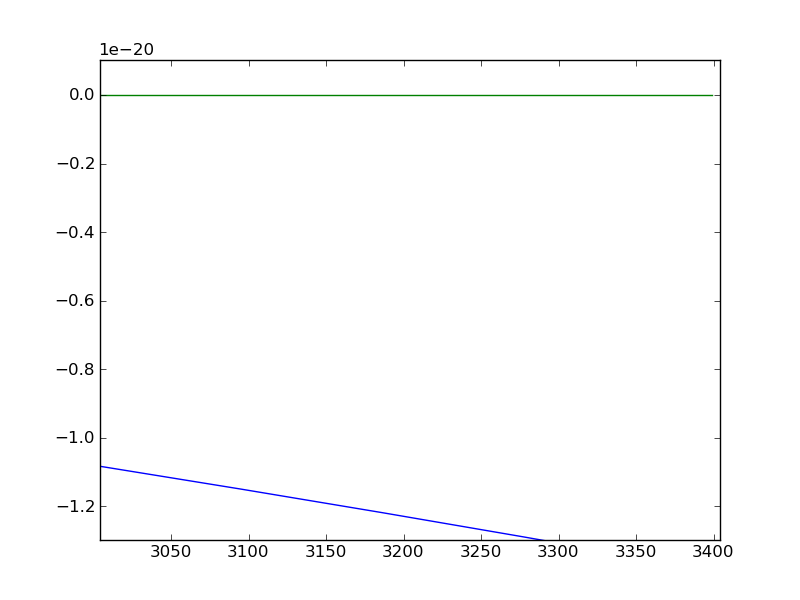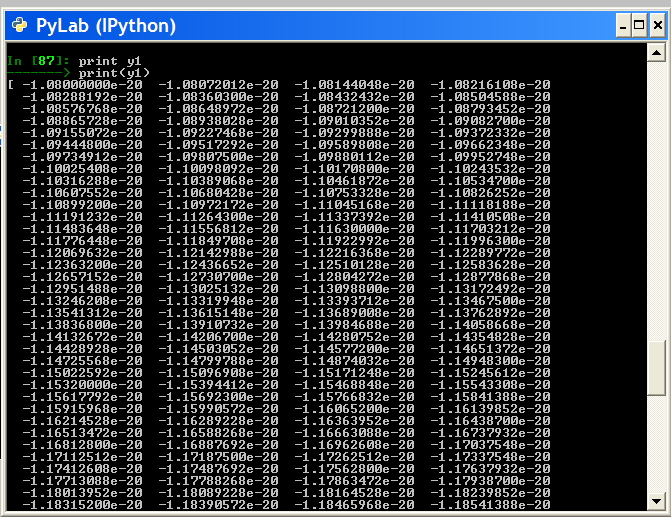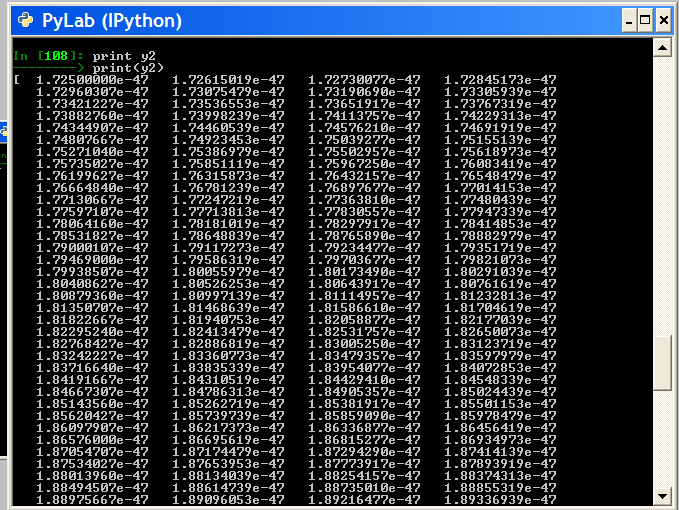···

On Tue, Jun 8, 2010 at 4:35 PM, Benjamin Root <ben.root@…1304…> wrote:

Waléria,

I repeat, you need to add parentheses for the denominator of that quantity in order to match what you wrote out on paper. You are missing a set of parentheses that is causing (3e14**2) to be multiplied rather than divided. This explains the huge values you are getting.

Ben Root

On Tue, Jun 8, 2010 at 2:05 PM, Waléria Antunes David <waleriantunes@…287…> wrote:

no, I think, did not understand my functions…see…i made a change my second function in the attached.

On Tue, Jun 8, 2010 at 3:48 PM, Benjamin Root <ben.root@…83…1304…> wrote:

I think I found your errors.
First, you are correct, the scientific notation in python is as you understand, there is nothing wrong with that.
Instead, you have a problem with order of operations. In the last quantity, you have "((x2)/4*(1e42)(3e142))"
According to the equation that you wrote out in the attached image, you need: "((x
2)/*(4(2e42)
(3e142)))"
Note that you needed parentheses for the entire denominator. Also, the 1e4
2 should have been 2e4**2.

This gives me values between 4.3125e-48 and 5.5359e-48.

Ben Root

On Tue, Jun 8, 2010 at 12:47 PM, Waléria Antunes David <waleriantunes@…287…> wrote:

``````I understand what you are saying, but my functions are these, attached.

Scientific notation in Python is not so?
**Math**               **Python**
``````

Pinc = 10-6 Pinc = 1e-6

3,0x1014 3e14

?

Then, changing my functions of the images attached for python is as bellow:

``````y1 = -108*(x**2)/(3e14**2)

y2 = 1*((1.38e-23*(1e0+4)/1e-6)*((x**2)/4*(1e4**2)*(3e14**2))))

``````

Is not it?

On Tue, Jun 8, 2010 at 1:34 PM, Gökhan Sever <gokhansever@…287…> wrote:

On Tue, Jun 8, 2010 at 10:19 AM, Waléria Antunes David <waleriantunes@…287…> wrote:

Hello!!!

My name is Waleria. I
work at INPE in Sao Jose dos Campos, Brazil. And I’d like to make a question. I’m in trouble to generate a two functions graph.

I have a problem to generate a graph of the two functions. I have this functions, is bellow:

``````__y1 = -108*(x**2)/(3e14**2)__
__y2 = 1*((1.38e-23*(1e0+4)/1e-6)*((x**2)/4*(1e4**2)*(3e14**2))))__

``````

You might need to check your y2. You are mixing integers and floats which possible have resulted with some rounding errors. I get e+30 when I assert the terms as floats in y2.

For the plotting: y1 is around -e-20 whereas y2 goes up to e+30. You can’t see the trend easily on one axes even if you could scale them logarithmically (hint logarithm is only defined for positive numbers). You can make a positive assumption for the y1 and plot them on one y-axes

yscale(‘log’)

axis(ymin=1e+20, ymax=1e+30)

Still this won’t let you see the functions trends that you are looking to see. I would suggest you to use multipe figures or investing a bit more time to use parasite axes example of JJ.

Gökhan

ThinkGeek and WIRED’s GeekDad team up for the Ultimate

GeekDad Father’s Day Giveaway. ONE MASSIVE PRIZE to the

lucky parental unit. See the prize list and enter to win:

http://p.sf.net/sfu/thinkgeek-promo

Matplotlib-users mailing list

Matplotlib-users@lists.sourceforge.net

https://lists.sourceforge.net/lists/listinfo/matplotlib-users

Did you try the example I posted?

Alan Isaac

···

On 6/9/2010 9:12 AM, Wal�ria Antunes David wrote:

Is there a way to plot my functions in the graph so that one can to visualize the difference between them.

Is there a way to plot my functions in the graph so that one can to

visualize the difference between them.

Did you try the example I posted?

Alan Isaac

Hello,

Alan Isaac,

Sure, i tried your example, but really i don’t was understanding with my graph, what was happening to my graph. So, i talked with my boss and he explained that was problem of scale.

I think i resolved my problem, if i get lost i write again for you…ok?

thank you very much

and sorry, i’m brazilian and i’m studying english and it is not very good…

···

On 6/9/2010 9:12 AM, Wal?ria Antunes David wrote:

On Wed, Jun 9, 2010 at 10:12 AM, Waléria Antunes David <waleriantunes@…287…> wrote:

Hello…

Benjamin,

I made a correction in my functions, i added parentheses for the denominator in the functions, saw the images in the attached:

two_functions.jpg
result in graph = images_.png
output values = values of the first function and values of the second function

Is there a way to plot my functions in the graph so that one can to visualize the difference between them.

This is correct my functions?

On Tue, Jun 8, 2010 at 4:35 PM, Benjamin Root <ben.root@…1304…> wrote:

Waléria,

I repeat, you need to add parentheses for the denominator of that quantity in order to match what you wrote out on paper. You are missing a set of parentheses that is causing (3e14**2) to be multiplied rather than divided. This explains the huge values you are getting.

Ben Root

On Tue, Jun 8, 2010 at 2:05 PM, Waléria Antunes David <waleriantunes@…2015…87…> wrote:

no, I think, did not understand my functions…see…i made a change my second function in the attached.

On Tue, Jun 8, 2010 at 3:48 PM, Benjamin Root <ben.root@…83…1304…> wrote:

I think I found your errors.
First, you are correct, the scientific notation in python is as you understand, there is nothing wrong with that.
Instead, you have a problem with order of operations. In the last quantity, you have "((x2)/4*(1e42)(3e142))"
According to the equation that you wrote out in the attached image, you need: "((x
2)/*(4(2e42)
(3e142)))"
Note that you needed parentheses for the entire denominator. Also, the 1e4
2 should have been 2e4**2.

This gives me values between 4.3125e-48 and 5.5359e-48.

Ben Root

On Tue, Jun 8, 2010 at 12:47 PM, Waléria Antunes David <waleriantunes@…287…> wrote:

``````I understand what you are saying, but my functions are these, attached.

Scientific notation in Python is not so?
**Math**               **Python**
``````

Pinc = 10-6 Pinc = 1e-6

3,0x1014 3e14

?

Then, changing my functions of the images attached for python is as bellow:

``````y1 = -108*(x**2)/(3e14**2)

y2 = 1*((1.38e-23*(1e0+4)/1e-6)*((x**2)/4*(1e4**2)*(3e14**2))))

``````

Is not it?

On Tue, Jun 8, 2010 at 1:34 PM, Gökhan Sever <gokhansever@…287…> wrote:

On Tue, Jun 8, 2010 at 10:19 AM, Waléria Antunes David <waleriantunes@…287…> wrote:

Hello!!!

My name is Waleria. I
work at INPE in Sao Jose dos Campos, Brazil. And I’d like to make a question. I’m in trouble to generate a two functions graph.

I have a problem to generate a graph of the two functions. I have this functions, is bellow:

``````__y1 = -108*(x**2)/(3e14**2)__
__y2 = 1*((1.38e-23*(1e0+4)/1e-6)*((x**2)/4*(1e4**2)*(3e14**2))))__

``````

You might need to check your y2. You are mixing integers and floats which possible have resulted with some rounding errors. I get e+30 when I assert the terms as floats in y2.

For the plotting: y1 is around -e-20 whereas y2 goes up to e+30. You can’t see the trend easily on one axes even if you could scale them logarithmically (hint logarithm is only defined for positive numbers). You can make a positive assumption for the y1 and plot them on one y-axes

yscale(‘log’)

axis(ymin=1e+20, ymax=1e+30)

Still this won’t let you see the functions trends that you are looking to see. I would suggest you to use multipe figures or investing a bit more time to use parasite axes example of JJ.

Gökhan

ThinkGeek and WIRED’s GeekDad team up for the Ultimate

GeekDad Father’s Day Giveaway. ONE MASSIVE PRIZE to the

lucky parental unit. See the prize list and enter to win:

http://p.sf.net/sfu/thinkgeek-promo

Matplotlib-users mailing list

Matplotlib-users@lists.sourceforge.net

https://lists.sourceforge.net/lists/listinfo/matplotlib-users